# Statistics: Basic Statistics IMany courses require that students in undergraduate degree programs have a basic understanding of descriptive statistics. Descriptive statistics are statistics that collect, summarize, classify and present data. This guide gives you an overview of one type of descriptive statistics, measures of variability.

Measures of variability are measures that allow you to determine the degree of variation within a population or sample, determine how representative a particular score is of a data set, and determine the scope and validity of any generalizations you wish to make based on your research observations. The measures of variability discussed in this handout are:

• Range
• Variance
• Standard Deviation

The range is the difference between the highest and lowest scores in a distribution. It is calculated by subtracting the lowest score from the highest score.

When is the range useful?
The range gives you a rough guide to the variability in a data set, as it tells you how a particular score compares to the highest and lowest scores.

EXAMPLE

14 40 42 47 49 51 71 81

(81 - 14) = 67

The range for this distribution would be (81 – 14) = 67. However, as you  can see, 14 is an outlier and skews this distribution because, if it were not in the distribution, the range would be (81 – 40) = 41.

HOW ELSE IS THE RANGE USEFUL

The range gives you a rough guide to the variability in a data set, as it tells you how a particular score compares to the highest and lowest scores within a data set. The range gives you only a limited amount of information, as data sets which are skewed towards a low score can have the same range as data sets which are skewed towards a high score, or those which cluster around some central score.

The sum of squares is a measure of variance or deviation from the mean.

It is calculated by summing of the squares of each score’s difference from the mean. The total sum of squares is another sum of squares; it considers not only the sum of squares from the factors, but also from randomness or error because the squaring of each score rids the equation of negative numbers. As you can see in the above example showing how to obtain the variance, step 5 requires you to find the sum of squares (SS).

EXAMPLE

a. Equation:b. Set of data: 2, 4, 6, 8

c. Square each data point and add them together: 22 + 42 + 62 + 82 = 4 + 16 + 36 + 64 = 120

d. Add together all of the data and square this sum: (2 + 4 + 6 + 8)2 = 400

e. Divide this by the number of data points to obtain 400/4 =100

f. We now subtract this number from 120: 120 - 100 = 20

g. This gives us that the sum of the squared deviations is 20.

The standard deviation is the square root of the variance.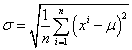Unlike the variance, the standard deviation is measured in the same units as the raw scores themselves; therefore, one cannot just use the variance. This is what makes the standard deviation more meaningful. For example, it would make more sense to discuss the variability of a set of IQ scores in IQ points than in squared IQ points because they would not be congruent with the score's meaning.

VariablesExample

Data Set: 2, 4, 4, 4, 5, 5, 7, 9

Find the mean:Calculate the deviations of each data point from the mean, and square the result of each:The variance is the mean of these values:Standard deviation is equal to the square root of the variance: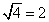Variance is the degree to which scores vary from their mean. The variance uses every score in the data set. The variance is calculated  by getting the average of the squared deviations from the mean.

VARIANCE EQUATION

Population Size                      Sample Sizeor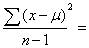To calculate the variance for a set of quiz scores:

1. Find the mean (M).

2. Find the deviation of each raw score from the mean (D).  To do this, subtract the mean from each raw score. To check your calculations, sum the deviation scores. This sum should be equal to zero. **Note that deviation scores below the mean will be negative.

3. Square the deviation scores (SS). We do this because by squaring the scores, negative scores are made positive and extreme scores are given relatively more weight.

4. Find the sum of the squared deviation scores.

5. Divide the sum by the number of scores. This yields the average of the squared deviations from the mean, or the variance Range.



EXAMPLE

Example Population Size:

Data Set: 5, 6, 7, 11, 12, 12, 13, 14, 18, 19, 21, 21, 22, 24, 35, 35, 50

Step 1: Find the meanStep 2 and 3: Find the deviation (D) from each score and square the deviation scores (SS).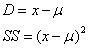Deviation Sum of Squares 5 - 20 = (-15) (-15)2 = 225 6 - 20 = (-14) (-14)2 = 196 7 - 20 = (-13) (-13)2 = 169 11 - 20 = (-9) (-9)2 = 81 12 - 20 = (-8) (-8)2 = 64 12 - 20 = (-8) (-8)2 = 64 13 - 20 = (-7) (-7)2 = 49 14 - 20 = (-6) (-6)2 = 36 18 - 20  = (-2) (-2)2 = 4 19 - 20 = (-1) (-1)2 = 1 21 - 20 = 1 12 = 1 21 - 20 = 1 12 = 1 22 - 20 = 2 22 =4 24 - 20 = 4 42 = 16 35 - 20 = 15 152 = 225 35 - 20 = 15 152 = 225 35 - 20 = 15 152 = 225 50 - 20 = 30 302 = 900

Step 4: Find the SumStep 5: Find the varianceThe variance is 138.11

TRY THIS EXERCISE

Using the steps above, find the variance for the following data set.

1.    8 11 12 14 17 17 18 19 22 29 35 38

 Data Set:  8 11 12 14 17 17 18 19 22 29 35 38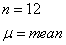Step 1: Find the meanStep 2 and 3: Find the Deviation and Sum of Squares     Deviations                                  Sum of Squares (8 – 20) = -12                     (-12)2 = 144 (11 – 20) = -9                     (-9)2 = 81                 (12 – 20) = -8                     (-8)2 = 64 (14 – 20) = -6                     (-6)2 = 36 (17 – 20) = -3                     (-3)2 = 9 (17 – 20) = -3                     (-3)2 = 9 (18 – 20) = -2                     (-2)2 = 4 (19 – 20) = -1                     (-1)2 = 1 (22 – 20) = 2                       22 = 4 (29 – 20) = 9                       92 = 81 (35 – 20) = 15                    152 = 225 (38 - 20) = 18                      182 = 324

Step 4: Find the sum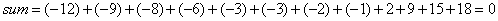Step 5: Find the variance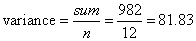DEFINITIONS

Measures of Variability: Measures that allow you to determine the degree of variation within a population or sample, determine how representative a particular score is of a data set, and determine the scope and validity of any generalizations you wish to make based on your research observations.

Range: The range is the difference between the highest and lowest scores in a distribution.

Variability/Variance: Degree to which the scores vary from their mean.

Standard Deviation: Square root of the variance.

Sum of Squares: The sum of squares is a measure of variance or deviation from the mean. It is calculated by summing the squares of each score’s difference from the mean. It is the sum of squared deviations.

Outlier: A data point that is distinctly separate from the rest of the data.